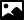Data Category ihm_2dem_class_average_fitting

GeneralCategory name
ihm_2dem_class_average_fitting
Required in PDB entries
no
Category group membership
ihm_group
Used in current PDB entries
No

Category Relationship DiagramsView full category relationship diagram including all dictionary data categories

Category Description```               Data items in the IHM_2DEM_CLASS_AVERAGE_FITTING category records the
details of the fitting of the model to the 2DEM class averages
used in the IHM modeling. The following conventions are recommended
while generating the rotation matrix and translation vector for
transformation.

- The model is rotated and translated to fit to the 2DEM image.
- The 2DEM image should be in the XY plane.
- The lower left image corner (image pixel index 0,0) should be at x,y,z = (0,0,0).
- The 2D image is scaled by the _ihm_2dem_class_average_restraint.pixel_size_width
and _ihm_2dem_class_average_restraint.pixel_size_height from the
IHM_2DEM_CLASS_AVERAGE_RESTRAINT table.
- The transformation is applied after the scaling and hence the translation vector
should account for the scaling.
- There are no specifications for Z translations i.e., how far the image should be
from the model while projecting. It may be set to zero.```

Category Example

```
_ihm_2dem_class_average_fitting.id                              1
_ihm_2dem_class_average_fitting.restraint_id                    1
_ihm_2dem_class_average_fitting.model_id                        1
_ihm_2dem_class_average_fitting.cross_correlation_coefficient   0.9
_ihm_2dem_class_average_fitting.rot_matrix                1.0
_ihm_2dem_class_average_fitting.rot_matrix                0.0
_ihm_2dem_class_average_fitting.rot_matrix                0.0
_ihm_2dem_class_average_fitting.rot_matrix                0.0
_ihm_2dem_class_average_fitting.rot_matrix                1.0
_ihm_2dem_class_average_fitting.rot_matrix                0.0
_ihm_2dem_class_average_fitting.rot_matrix                0.0
_ihm_2dem_class_average_fitting.rot_matrix                0.0
_ihm_2dem_class_average_fitting.rot_matrix                1.0
_ihm_2dem_class_average_fitting.tr_vector                    0.0
_ihm_2dem_class_average_fitting.tr_vector                    0.0
_ihm_2dem_class_average_fitting.tr_vector                    0.0```